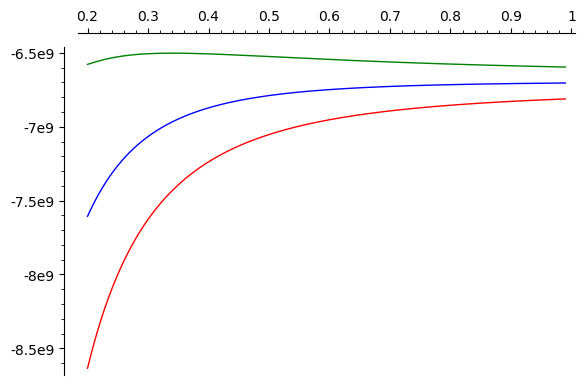# when plot()ing, where can i call .lower() and .upper() in order to get the band of uncertainty?

when i plot a function with parameters with and upper and lower value like this:

p1=plot( v_ges_rhs.subs({
d_e : RIF(.999,1.001),
x : -.25,
d_m : RIF(.05,.15),
e_m : e_0 * RIF(80.5,81.5),
e_s : e_0 * RIF(3,4)
}), ( d_k, 0.2, .99 ) )


at what point can i call .lower() or .upper() to get the lower or upper boundary of my function? I would then overlay both into one graph for visualisation of the error.

edit retag close merge delete

Sort by » oldest newest most votedLet's define

idk = v_ges_rhs.subs({
d_e : RIF(.999,1.001),
x : -.25,
d_m : RIF(.05,.15),
e_m : e_0 * RIF(80.5,81.5),
e_s : e_0 * RIF(3,4)
})


This is a symbolic expression containing RIF numbers and the only symbolic variable is d_k.

At any point in the interval we can evaluate it to a symbolic expression which is just a RIF number, e.g.

sage: idk.subs({d_k : 0.2})
-1.?e10


Unfortunately we cannot call .upper() on this directly, because there is still a symbolic wrapper around it:

sage: type(idk.subs({d_k : 0.2}))
<type 'sage.symbolic.expression.Expression'>


To remove the wrapper, we use conversion to RIF:

sage: RIF(idk.subs({d_k : 0.2}))
-1.?e10
sage: RIF(idk.subs({d_k : 0.2})).upper()
-6.57758536841850e9
sage: RIF(idk.subs({d_k : 0.2})).lower()
-8.63461563768822e9


We can define functions which do this at a given point

f = lambda x: idk.subs({d_k: x})
f_lower = lambda x: RIF(idk.subs({d_k : x})).lower()
f_upper = lambda x: RIF(idk.subs({d_k : x})).upper()


and plot them:

plot([f, f_lower, f_upper],(x,0.2,0.99), color=['blue', 'red', 'green'])more

1